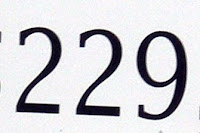## Wednesday, July 29, 2009

### 229

229 is a prime number.

227 and 229 form a twin prime pair.

229 has a representation as a sum of two squares: 229 = 22 + 152.

229 is the hypotenuse of a primitive Pythagorean triple: 2292 = 602 + 2212.

The sum of the first
229 primes divides the product of the first 229 primes.

229 is the smallest prime that remains prime when added to its reversal: 229 + 922 = 1151.

229 is the difference between 33 and 44.229 is the highest you can score in a standard game of bowling if your score in each of the ten frames is required to be a prime number.

Source:
Prime Curios!